Email Us: info@lupinepublishers.comCall Us: +1 (914) 407-6109   57 West 57th Street, 3rd floor, New York - NY 10019, USA

# Lupine Publishers Group

## Lupine Publishers

Submit Manuscript

ISSN: 2644-1381# The T-R {Generalized Lambda V} Families of Distributions Volume 1 - Issue 2

Received: November 11, 2018;   Published: December 06, 2018

DOI: 10.32474/CTBB.2018.01.000108

## Abstract

The four-parameter generalized lambda distribution (GLD) was proposed in . We say the GLD is of type V, if the quantile function corresponds to Case(v) in of , that is,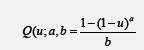where u 2 (0, 1) and a, b 2 (−1, 0). In this short note, we introduce the T-R {Generalized Lambda V} Families of Distributions and show a sub-model of this class of distributions is significant in modeling real life data, in particular the Wheaton river data, . We conjecture the new class of distributions can be used to fit biological and health data.

Keywords and Phrases: Generalized Lambda Distribution; Exponential Distribution; Weibull Distribution

## Contents

a) The T – R {Y} Family of Distributions

b) The New Distribution

c) Practical Significance

d) Open Problem

### The T – R {Y} Family of Distributions

This family of distributions was proposed in . In particular, let T, R, Y be random variables with CDF’s FT (x) = P (T _ x), FR(x) = P (R _ x), and FY (x) = P (Y _ x), respectively. Let the corresponding quantile functions be denoted by QT (p), QR(p), and QY (p), respectively. Also, if the densities exist, let the corresponding PDF’s be denoted by fT (x), fR(x), and fY (x), respectively. Following this notation, the, the CDF of the T – R {Y} is given by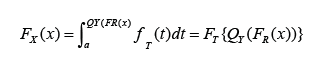and the PDF of the T-R{Y} family is given by### The New Distribution

Theorem: The CDF of the T-R {Generalized Lambda V} Families of Distributions is given bywhere the random variable R has CDF FR(x), the random variable T with support (0,1) has CDF FT, and a, b 2 (−1, 0) Proof. Consider the integral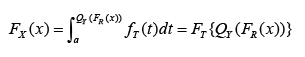and let Y follow the generalized lambda class of distributions of type V, where the quantile is as stated in the abstract

Remark: the PDF can be obtained by differentiating the CDF

### Practical Significance

In this section, we show a sub-model of the new distribution defined in the previous section is significant in modeling real life data. We assume T is standard exponential so that FT (t) = 1 − e−t, t > 0 and R follows the two-parameter Weibull distribution, so that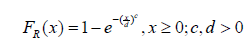Now from Theorem 2.1, we have the following

Theorem: The CDF of the Standard Exponential-Weibull {Generalized Lambda V}

Families of Distributions is given by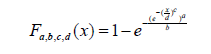where c, d > 0 and a, b 2 (−1, 0)

By differentiating the CDF, we obtain the following

Theorem: the PDF of the Standard Exponential-Weibull {Generalized Lambda V} Families of Distributions is given by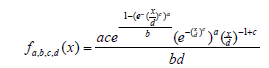where c, d > 0 and a, b 2 (−1, 0)

Remark: If a random variable B follows the Standard Exponential-Weibull {Generalized

Lambda V} Families of Distributions write

B _ SEWGLV (a, b, c, d)

### Open Problem

Conjecture: The new class of distributions can be used in forecasting and modelingn of biological and health data. Related to the above conjecture is the following

Question: Is there a sub-model of the T-R {Generalized Lambda V} Families of Distributions that can fit?  (Appendix 1) and (Figures 1-3).

### Appendix 1: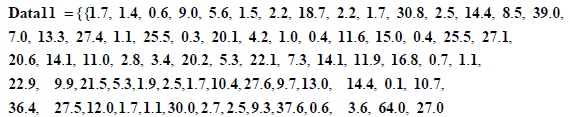DataQ1 = Flatten Data11; Length DataQ1 72

Min DataQ1 0.1; Max DataQ1 64.

AX1 = Empirical Distribution DataQ1

Data Distribution «Empirical», { 58}

K1 = Discrete Plot [CDF [AX1, x ], {x, 0, 65, (65-0)/58} , Plot Style c {Black, Thick} , Plot Markers c {Automatic, Small} , Filling c None,

Plot Range c All]

(Figure 1)

F1 x_, a_, b: = 1 - 1 - x ^ ab

CDF Weibull Distribution c, d, x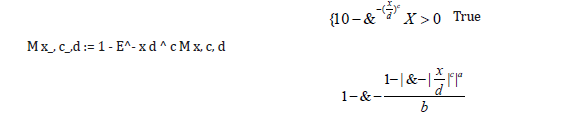### I. Weibull. nb

D 1 - E^ - F1 M x, c, d, a, b, x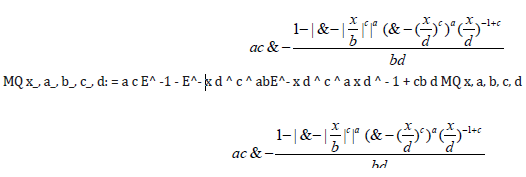PLK1 = Sum Log MQ DataQ1, a, b, c, di, i,1, Length DataQ1;

JK=D [PLK, a];

JK1=D [PLK, b];

JK2=D [PLK, c];

JK3=D [PLK,d];

Find Root JK, JK1, JK2, JK3, a, - 0.11 ,b, - 0.12 , c, 0.9 , d, 11.6

a c – 7.2577, b c – 0.395297, c c 0.776499, d c 657.998

RR = Plot 1 - E^ - F1 M x, 0.776499, 657. 998, - 7.2577, - 0.395297, x, 0, 65, Plot Style c Thick, Blue, Plot Range c All

(Figure 2)

II. Weibull. nb

CMU = Show K1, RR, Plot Range c All

(Figure 3)

Export “CMU.jpg”, CMU CMU.jpg

Close## Online Submission System

### Submit ManuscriptClick here for Manuscript Sample Templates### Drag and drop files here

or

( For multiple files submission, zip them in a single file to submit. For file zipping software Download )